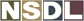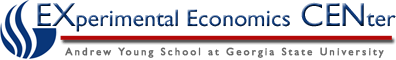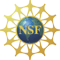Students / Subjects# Calculating Inflation with Price Indexes

Inflation is calculated by taking the price index from the year in interest and subtracting the base year from it, then dividing by the base year.  This is then multiplied by 100 to give the percent change in inflation.

Inflation = (Price Index in Current Year – Price Index in Base Year) * 100

Price Index in Base Year

From our previous example:

Inflation =  (120-100) *  100 = 0.2 * 100 = 20%

100

Thus from 2006 to 2007, inflation has risen 20%.

In this context, inflation is measured as a percentage change in the price index from one period to the next.

If the percentage change in the price index is negative it shows deflation rather than inflation.

Back to Price Index

Back to Inflation